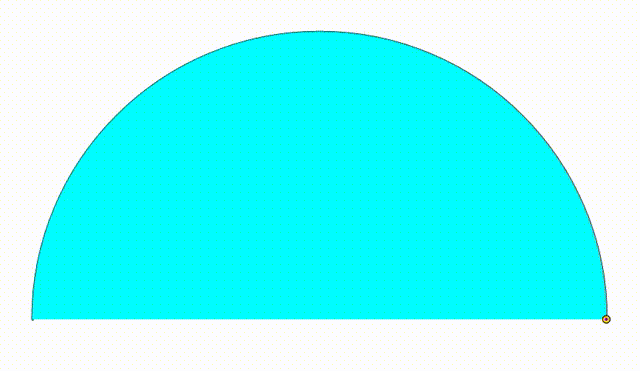# Dynamic Geometry: P94The diagram shows a black semicircle. The cyan and green semicircles are tangent to each other and internally tangent to the black semicircle. They are growing and shrinking freely so that the sum of their radius is always equal to the black semicircle's radius. We draw a red vertical segment using their tangency point. At last we inscribed two yellow circles so they are tangent to the red line, to the black semicircle and to one of the two bottom semicircles. The center of each yellow circle and the tangency point between the yellow circles and the red line are used to draw a purple quadrilateral. When the area of the purple quadrilateral is maximum, the ratio of the larger semicircle's radius to the radius of the smaller semicircle can be expressed as:

$\dfrac{p+q\sqrt{m}}{n}$

where $p$, $q$, $m$ and $n$ are coprime positive integers and $m$ is square-free. Find $p+q+m+n$.

×